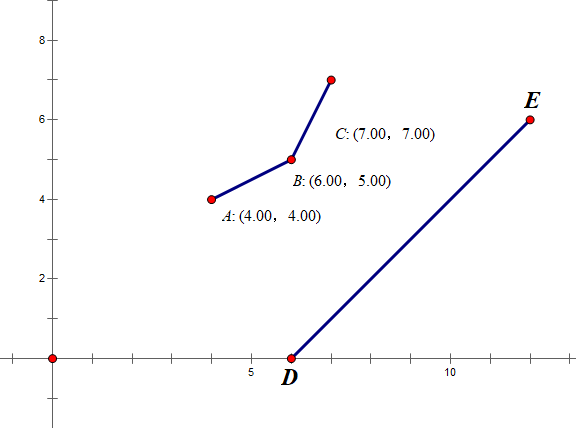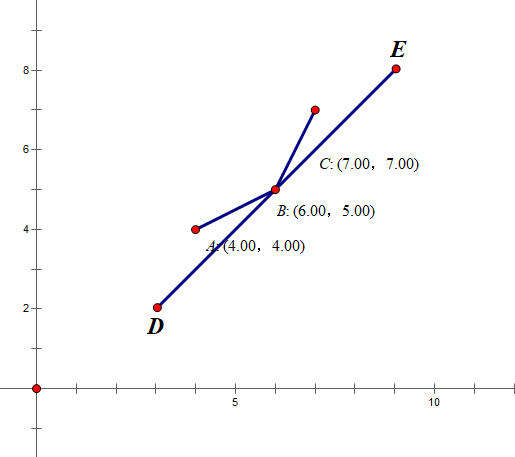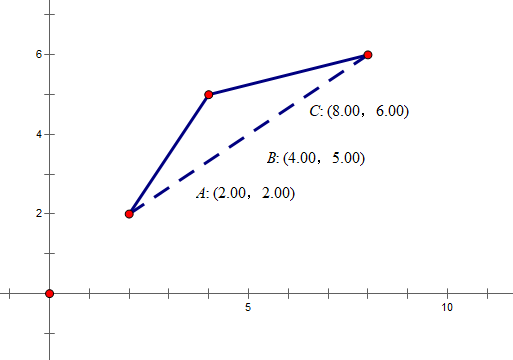# 洛谷 P2365/POJ 1180 任务安排 题解【斜率优化DP】【单调队列】【费用提前计算】

斜率优化入门。

## 题目描述

N个任务排成一个序列在一台机器上等待完成（顺序不得改变），这N个任务被分成若干批，每批包含相邻的若干任务。从时刻0开始，这些任务被分批加工，第i个任务单独完成所需的时间是Ti。在每批任务开始前，机器需要启动时间S，而完成这批任务所需的时间是各个任务需要时间的总和（同一批任务将在同一时刻完成）。每个任务的费用是它的完成时刻乘以一个费用系数Fi。请确定一个分组方案，使得总费用最小。

5
1
1 3
3 2
4 3
2 3
1 4

153

## 题解：

对于5000的数据，我们只需要费用提前计算一下，每分一段就把后面所有任务的等待时间全部加上，然后$n^2$遍历转移一下就可以了。对于10000+的数据，大多数评测机$O(n^2)$都过不了了。这个题可以通过斜率优化，来优化到$O(N)$。

首先，$n^2$的做法，列出状态转移方程，也就是预处理出时间前缀和bt，费用系数前缀和bc，因为任务的完成是连续的。因此，在每次分段时，利用费用提前计算，就不用枚举前面经过了多少个分段了。

### $f[i]=\min\limits_{0\le j\le i}\{ f[j]+s\times (bc[n]-bc[j])+bt[i]\times bc[i]-bc[j]\}$

那么我们如果把这个式子中的min去掉，变为一个函数求最小值问题，可不可行呢？我们把f[j]挪到左边去，得到

### $f[j]=(s+bt[i])\times bc[j]+f[i]-bc[i]\times bt[i]-s\times bc[n]$

此时要求f[j]的最小值，就要算出右边的函数。而其中$f[i]-bc[i]\times bt[i]-s\times bc[n]$与j无关，因此可以视作一个常数$b$，而自变量是$bc[j]$，斜率为$k=s+bt[i]$。要让f[j]最小，就转变为了一个线性规划的问题：恒定斜率，求出最小截距，也就是$f[i]-bc[i]\times bt[i]-s\times bc[n]$。

这样一来，我们要为每一次决策找到最优方案，符合上述直线的点坐标为$(bc[j],f[j])$，也就是要使截距最小。对此，我们可以稍作分析：可以发现，在这个图中，DE是满足斜率为上述条件的直线，B点是第一个符合要求的点。接下来分析这样的点有什么特点。

首先，如果有一个点会在某些斜率下被第一个找到，他一定在下凸壳上。也就是说，所有凸壳上的点都可能成为最优解。不过也会有一些不合法的情况，比如右图，A点就是不合法的，因为它不能转移到当前直线表示的状态；所有在B点左侧的点都要舍掉，那么可以发现，在B点左边的点有一个特点：它们所构成的凸壳，边的斜率都比DE的斜率要小。而在原始的状态转移方程中，可以看出f[i]一定是单调增的，所以之后的状态也就只能从B点右侧的点转移了。

同时，为了保证凸壳，边界上的点不能上凸，像这样的点B就是不合法的：因此就要像Graham-Scan算法一样，把每次合法的点放在一个栈里，直到符合栈中只剩一个点或者栈顶两个点与当前点为下凸关系。

综上所述，一个满足既要排除较小点，又要退出较大点的数据结构，单调队列是在适合不过的了。

在单调队列里，两点间斜率一定是递增的，因为维护了一个凸壳。而每次把不合法情况剔除完后，队首的点一定是最优的，也就是第一个满足条件的，因为剩下的都是斜率大于$k$的线，所以一条斜率为$k$的线扫上去，队首那个元素一定最先被扫到。等最后找到队尾所有与当前点为上凸关系的点，从后面出队就可以了。

## Code：

#include<cstdio>
#include<cstring>
int f,bc,bt;
int q,l=0,r=0;
int main()
{
int n,s;
scanf("%d%d",&n,&s);
for(int i=1;i<=n;i++)
{
scanf("%d%d",&bt[i],&bc[i]);
bt[i]+=bt[i-1];
bc[i]+=bc[i-1];
}
q[++r]=0;
for(int i=1;i<=n;i++)
{
while(r-l>1&&f[q[l+2]]-f[q[l+1]]<=(s+bt[i])*(bc[q[l+2]]-bc[q[l+1]]))//把斜率小于k的全部拉出去
l++;

f[i]=f[q[l+1]]+s*(bc[n]-bc[q[l+1]])+bt[i]*(bc[i]-bc[q[l+1]]);

while(r-l>1&&(f[i]-f[q[r]])*(bc[q[r]]-bc[q[r-1]])<=(f[q[r]]-f[q[r-1]])*(bc[i]-bc[q[r]]))//不满足下凸条件
r--;

q[++r]=i;
}
printf("%d\n",f[n]);
return 0;
}


### 说点什么Subscribe

/* */